## EXCEL YIELD function gives incorrect results

Apr 7

Written by: Charles Flock
4/7/2010 6:23 PMWherein we delve deep into EXCEL’s YIELD function and discover the inconsistencies between the YIELD function and the PRICE function in EXCEL.

The purpose of the YIELD function is to return a value for the yield of a bond, given the price of the bond, such that when passing the calculated YIELD value back to the PRICE function, it returns the supplied price. Another way of saying this is that the YIELD function satisfies the following condition:
PRICE(settlement, maturity, rate, YIELD(settlement, maturity, rate, price, redemption, frequency, basis), redemption, frequency, basis) – price = 0.
Today we will see that this is not always true in EXCEL. And, surprisingly enough, it’s not true in the simplest of cases, where the settlement date is in the final coupon period.
Bond math (or figuration) can make anybody’s eyes glaze over, so I will try to keep it simple (which, I will admit up front, is not my strong suit). The YIELD calculation is really two calculations, based upon how many coupon payments remain in the bond on the settlement date. If there is more than one coupon period, the YIELD is calculated by guessing; smart guessing, but guessing nonetheless. According to the EXCEL documentation, they use the Newton method of guessing, which, when properly implemented, is a very good method of guessing for a bond. The reason for using guessing is that the bond formula is a polynomial for which there is no closed form solution—which is simply an eye-glazing way of saying that you have to guess.
However, when a bond is in the final coupon period, the YIELD can be calculated without guessing. The formula is straightforward:
yield = (mat_amt / dirty_price - 1) * E / DSR * frequency
Where
mat_amt             the redemption amount plus the coupon interest payable at maturity
dirty_price          the price of the bond plus the accrued interest
E                         the number of days in the coupon period
DSR                     the number of days from the settlement date to the maturity date
frequency           the number of coupon payments in a year
Interestingly enough, if you refer to the EXCEL documentation (which can be found here), and you look at the equation they supply, there is no mention of the price. I don’t believe that this is the equation that they use (but feel free to try for yourself) because it implies that the price of the bond has no effect on the calculation of the yield. If that were the case, nobody could possibly be using the function. I am not trying to demonstrate that the YIELD calculation is always wrong; just sometimes.
In our first example (download spreadsheet here), we have a bond with a maturity date of 5/25/2010. The interest rate is zero, eliminating the need to calculate the interest due at maturity and the accrued interest. The bond has a redemption value of 100 (making it equal to the par value), pays interest twice a year, and has a basis code of zero, which EXCEL defines as US (NASD) 30/360. We have entered 4 settlement dates from 12/29/2009–01/01/2010.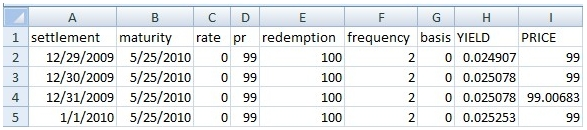In H2, we have entered the formula =YIELD(A2,B2,C2,D2,E2,F2,G2) and then copied the formula to H3:H5. In I2 we have entered the formula =PRICE(A2,B2,C2,H2,E2,F2,G2) and copied it to I2:I5. Since the supplied price in all cases is 99, the value supplied by YIELD should return a PRICE of 99. As you can plainly see, for the settlement date of 12/31/2009 it did not.
You should also note that YIELD returned the same value for settlement on the 12/30/2009 and settlement on 12/31/2009. We’ll come back to that after we look at another example.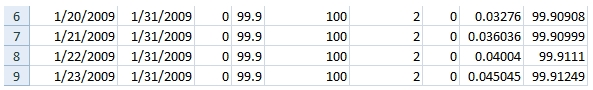Here, we have changed the maturity date to 1/31/2009, and the price to 99.9 and calculated the YIELD for the settlement dates from 1/20/2009 to 1/23/2009. We copied our YIELD formula to H6:H9 and our PRICE formula to I6:I9. In none of the four examples do we get the expected price of 99.9 when using the yield values produced by the EXCEL YIELD function.
Let’s see what happens if we use the yield formula described at the beginning of this blog. We calculate the number of days in the coupon period by entering =COUPDAYS(A2,B2,F2,G2) in J2 and copying it to J3:J9. We calculate the number of days from settlement date to maturity date by entering =COUPDAYSNC(A2,B2,F2,G2) in K2 and copying it to K3:K9.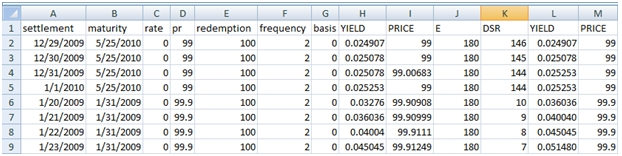In L2 we enter = (E2/D2-1)*J2/K2*F2 and copy that to L3:L9. This gives us the yield that we will then use in the PRICE function. We enter = PRICE(A2,B2,C2,L2,E2,F2,G2) into M2 and copy it to M3:M9. We can immediately see that the formula we entered in the K column returns that the price that had been input into the function. In other words, our manual calculation satisfies the requirements of the YIELD calculation.
So what is going on in EXCEL? In our first example, we can see that the EXCEL calculations of YIELD for settlement on 12/30/2009 and 12/31/2009 are the same (.025078), whereas as in our calculations of yield the values are the same for settlement on 12/31/2009 and 1/1/2010 (.025253). It seems safe to assume then that the values of E and DSR in the YIELD function are different from the corresponding values in the PRICE, COUPDAYS and COUPDAYSNC functions. If we assume that the value of E is correct (which seems like a safe assumption in this example), then we can conclude that it is the value of DSR (the number of days from settlement to redemption) that is incorrect in the EXCEL YIELD function.
Let’s look at the bond that matures on 1/31/2009. None of the YIELD function results was correct. If we examine the results closely, however, we can see that the YIELD function result for 1/21/2009 is the same as our calculation for 1/20/2009 and that, in fact, all of the built-in function results agree with our results from the previous settlement day. Again, this seems to point to the EXCEL calculation of DSR in the YIELD function as being incorrect (or at least inconsistent with the PRICE function).
Let’s look at a different problem with EXCEL’s YIELD function. We have added the following rows to our spreadsheet: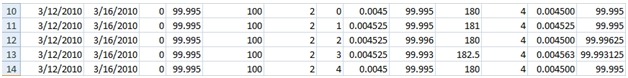We have a zero-coupon bond maturing on 3/16/2010, settling on 3/12/2010 at a price of 99.995 and we have used all 5 basis codes (0–4). As you can see, the PRICE calculated from YIELD for basis codes 2 and 3 does not agree with the inputted price. Even worse, the formula that we used in the previous examples also did not calculate the correct yield. In fact, in order to calculate the correct value for YIELD, we need to have the following values in DSR: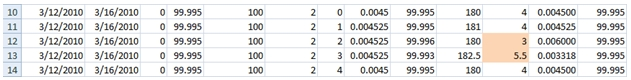Where did those values come from? In the case where the basis code is 2, the COUPDAYBS function calculates that there are 177 days from the previous coupon date to the settlement date. Since the coupon period is 180 days, the value for DSR (days from settlement to redemption) is 3 days, rather than the 4 days calculated by the COUPDAYSNC function. The same thing is true when the basis code is 3. There are 182.5 days in the coupon period; there are 177 days from the previous coupon date to the settlement date; if we calculate DSR as 182.5 – 177 then we get 5.5 days, which generates a yield value that returns the correct price.
We can change our spreadsheet by entering a new formula in the range K2:K14. In K2, enter =J2-COUPDAYBS(A2,B2,F2,G2) and then copy the formula to K3:K9. Now, all of our calculations of yield return the supplied price.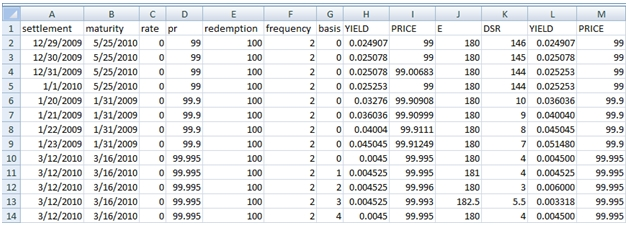It seems like the EXCEL YIELD function can be quite unreliable under certain conditions, which we have laid out here. Will you encounter these conditions in the real world? I can’t really say, but it’s certainly enough to make me wonder why EXCEL persists in having problems in simple functions like this.

 The point here is not whether or not the COUPDAYSNC function is correct, but in trying to ascertain what values the PRICE function requires in order to return the original price

Tags:
Categories:
Location: BlogsThe WestClinTech Blog

## Search Blogs

 KeywordsPhrase

## Blog Archives

Archive
 < September 2023 >
SunMonTueWedThuFriSat
272829303112
3456789
10111213141516
17181920212223
24252627282930
1234567
Monthly
Go

### SupportCopyright 2008-2023 Westclintech LLC         Privacy Policy        Terms of Service